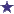## Comments for A Calculator for Polynomial Equations of Degree Five and Above

Average RatingRatingPoint of consideration by: Anonymous I would comment here as the author of this article that the general quintic can be reduced to the form x^5+ p = 0. In this case p = a4* x^4 + a3* x^3 + a2*x^2 + a1*x + a0. This may be necessary to enable using the general functions y= - x^5 and y = a4* x^4 + a3* x^3 + a2*x^2 + a1*x + a0 to help enable come with a general solution of a quintic. The two functions are graphed on the same axis and the points of intesection (p) are determined. The general solution of a quintic would be x = {-p}^(1/5) * e^(2* i*pie/5).

Return to A Calculator for Polynomial Equations of Degree Five and Above.